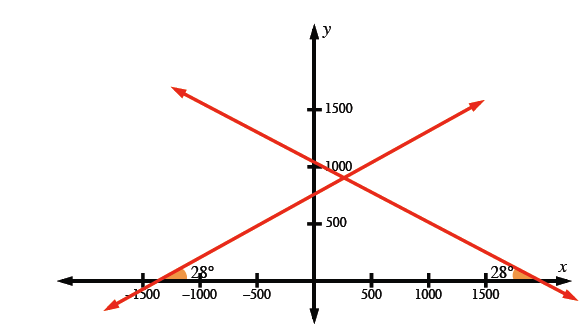### Home > PC > Chapter 6 > Lesson 6.2.1 > Problem6-77

6-77.

Find the equation of all possible lines that pass through the point $\left(285, 912\right)$ and make an angle of $28°$ with the $x$-axis. Hint: No arithmetic is required, but you do need your calculator.

Write the equation in the point slope form. Don't forget slope can be positive or negative.